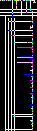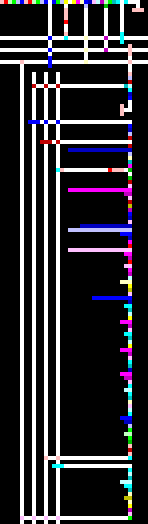# Factorial in Piet

Example for versions npiet 1.2

This example was generated automatically. The original program translated into the image follows; it uses iterative factorial calculation. Note that values of 13! and larger are wrong due to overflow.

``````main()
{
f = 1;
for ( i = 0; i <= 16; i++ )
{
__outn(i);
asm{ @"! = " }
__outn(f);
__out(10);
f = f * (i+1);
}
}
``````Factorial in Piet (autogenerated)Factorial in Piet (autogenerated, 4x scale)